Worksheets and No Prep Teaching Resources Math Second Grade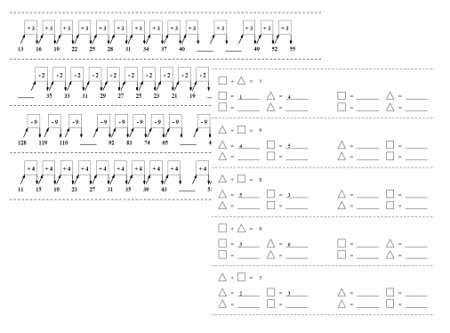Second Grade Algebra Printables, Worksheets, and Lessons

Second Grade Algebra Mixed Review Book

Skip Counting
Easy Skip Counting with Numberline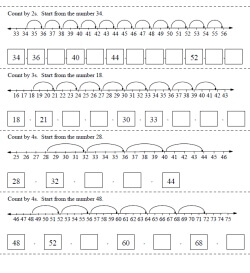Count by 2's, 3's, or 4's - addition Count by 5's - addition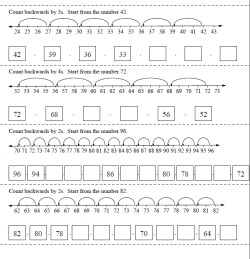Count by 2's, 3's, or 4's - subtraction Count by 5's - subtraction

Patterns
Circle Growing or Decreasing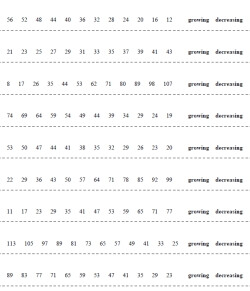Circle growing or decreasing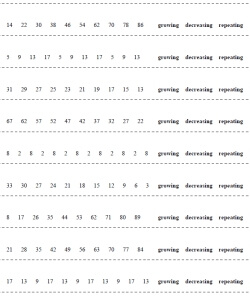Circle growing, decreasing, or repeating

Complete the Sequence - Change Boxes Given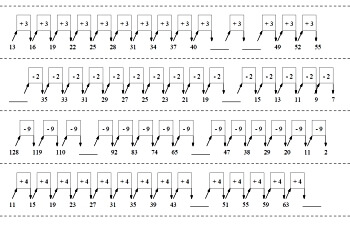Complete the pattern - all the change values are given - 2 blanks Complete the pattern - all the change values are given - 4 to 6 blanks

Complete the Sequence and Fill-In Change Boxes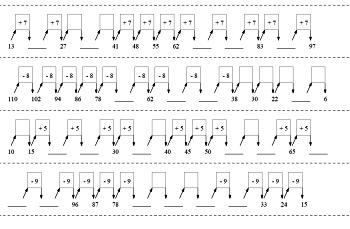Complete the pattern - 2 change values are blank - 2 blanks Complete the pattern - 2 to 4 change values are blank - 4 to 6 blanks

Mystery Numbers: Greater and Less Than
One Mystery Number with Number Line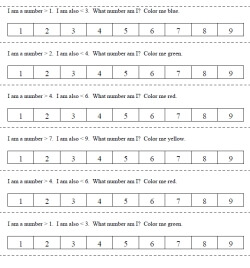Numbers 1 to 9: Missing Number - with Number Line - Color In (greater/less than signs always same order) Numbers 1 to 9: Missing Number - with Number Line - Color In (greater/less than signs in different orders)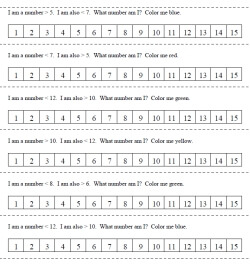Numbers 1 to 15: Missing Number - with Number Line - Color In (greater/less than signs always same order) Numbers 1 to 15: Missing Number - with Number Line - Color In (greater/less than signs in different orders)

One Mystery Number Fill-in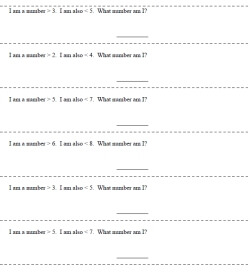Numbers 1 to 9: Missing Number - Fill-in - Color In (greater/less than signs always same order) Numbers 1 to 9: Missing Number - Fill-in - Color In (greater/less than signs in different orders)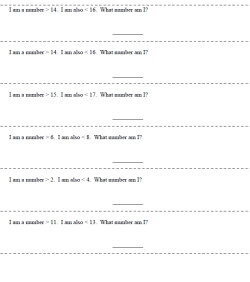Numbers 1 to 19: Missing Number - Fill-in - Color In (greater/less than signs always same order) Numbers 1 to 19: Missing Number - Fill-in - Color In (greater/less than signs in different orders)

One or More Mystery Numbers with Number Line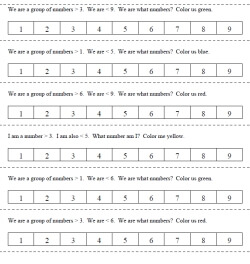Numbers 1 to 9: Missing Numbers - with Number Line - Color In (greater/less than signs always same order) Numbers 1 to 9: Missing Numbers - with Number Line - Color In (greater/less than signs in different orders)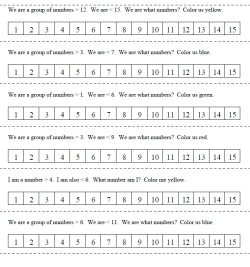Numbers 1 to 15: Missing Numbers - with Number Line - Color In (greater/less than signs always same order) Numbers 1 to 15: Missing Numbers - with Number Line - Color In (greater/less than signs in different orders)

One or More Mystery Numbers Fill-in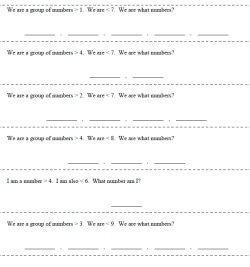Numbers 1 to 9: Missing Numbers - Fill-in - Color In (greater/less than signs always same order) Numbers 1 to 9: Missing Numbers - Fill-in - Color In (greater/less than signs in different orders)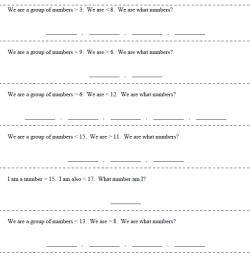Numbers 1 to 19: Missing Numbers - Fill-in - Color In (greater/less than signs always same order) Numbers 1 to 19: Missing Numbers - Fill-in - Color In (greater/less than signs in different orders)

Change in Numbers: How Did it Change?
Numbers 1-9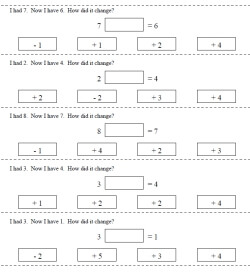Numbers 1 to 9 - the change will be from 1 to 3 - equation is given Numbers 1 to 9 - the change will be from 1 to 5 - equation is given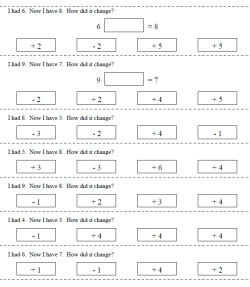Numbers 1 to 9 - the change will be from 1 to 3 - equation is given in HALF of the problems Numbers 1 to 9 - the change will be from 1 to 5 - equation is in HALF of the problems given

Making a Number Sentence: Unknowns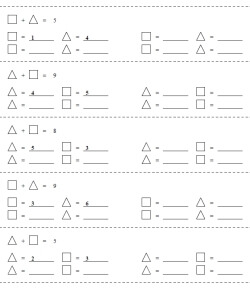Maximum sum of 9 - one answer given for each problem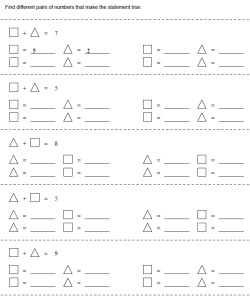Maximum sum of 9 - one answer given for first two problem

More Patterns

Patterns and Functions: IN and OUT
Easier Functions: The Rule is Given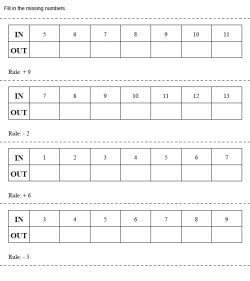Easy: Rule is given, IN are numbers that increase by 1, Fill in OUT, addition or subtraction, horizontal display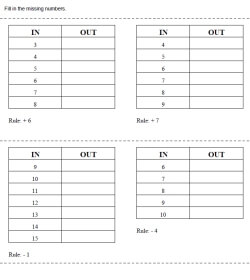Easy: Rule is given, IN are numbers that increase by 1, Fill in OUT, addition or subtraction, vertical display

Patterns and Functions: IN and OUT with Shapes
Easier Functions: The Rule is Given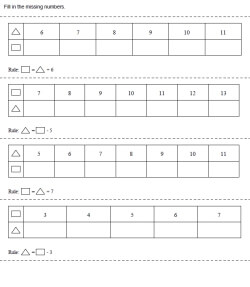Easy: Rule is given, IN are numbers that increase by 1, Fill in OUT, addition or subtraction, horizontal display

Sequences

Possible Number Solutions - Greater and Less Than - Number Sentences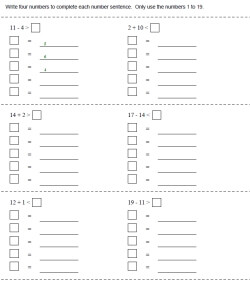Numbers to 9 (some answers given in first problem)

Ordered Pairs

Make the Scale Balance
Easier: Balance is Equal - Add the Missing Blocks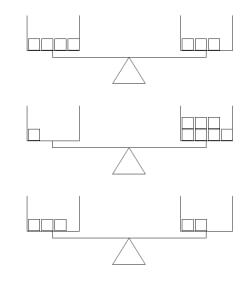Add the missing blocks Add the missing blocks and write the number of blocks on each side Add the missing blocks - one problem does not need any blocks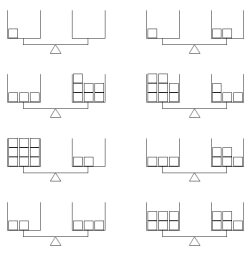More problems per page More problems per page - one problem does not need any blocks

One Side of Balance is Heavier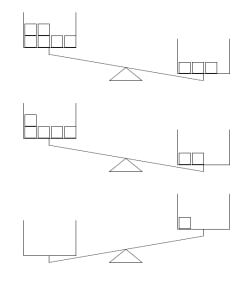Add the missing blocks Add the missing blocks and write the number of blocks on each side Add the missing blocks - one problem does not need any blocks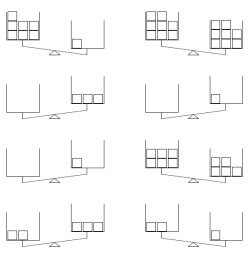More problems per page More problems per page - one problem does not need any blocks

Some Balances are Equal - Others have Heavier Sides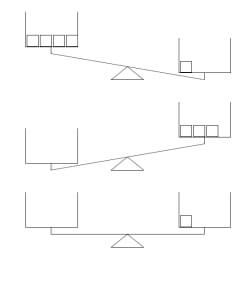Add the missing blocks Add the missing blocks and write the number of blocks on each side Add the missing blocks - one problem does not need any blocks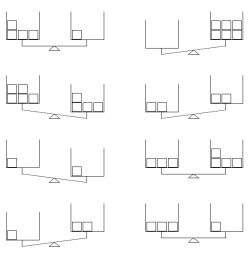More problems per page More problems per page - one problem does not need any blocks

Greater and Less Than: Make the Scale Balance
Easier: Maximum Sum of 19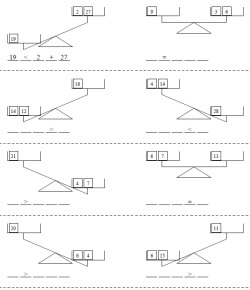Answer given for the first problem - the sign is given for all the problems Answer given for the first problem - the sign is given for the first four problems Answer given for the first problem No answers, but blank lines are shown for parts ___ ____ ____ ____ _____
Maximum Sum of 99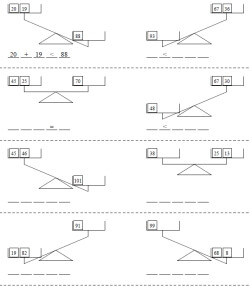Answer given for the first problem - the sign is given for all the problems Answer given for the first problem - the sign is given for the first four problems Answer given for the first problem No answers, but blank lines are shown for parts ___ ____ ____ ____ _____
Algebra Challenge - Maximum Sum of 19 - All balances are equal and there is one UNKNOWN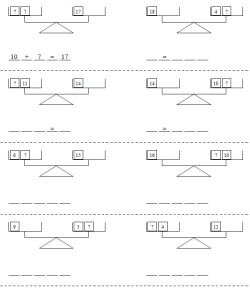Algebra Challenge: Answer given for the first problem - the sign is given for all the problems Algebra Challenge: Answer given for the first problem - the sign is given for the first four problems Algebra Challenge: Answer given for the first problem Algebra Challenge: No answers, but blank lines are shown for parts ___ ____ ____ ____ _____

Have a suggestion or would like to leave feedback?